# NCERT Solutions for Class 8 Maths Chapter 9 Algebraic Expressions and Identities Ex 9.5

NCERT Solutions for Class 8 Maths Chapter 9 Algebraic Expressions and Identities Ex 9.5 are part of NCERT Solutions for Class 8 Maths. Here we have given NCERT Solutions for Class 8 Maths Chapter 9 Algebraic Expressions and Identities Ex 9.5.

 Board CBSE Textbook NCERT Class Class 8 Subject Maths Chapter Chapter 9 Chapter Name Algebraic Expressions and Identities Exercise Ex 9.5 Number of Questions Solved 8 Category NCERT Solutions

## NCERT Solutions for Class 8 Maths Chapter 9 Algebraic Expressions and Identities Ex 9.5

Question 1.
Use a suitable identity to get each of the following products: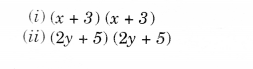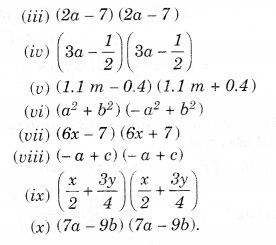Solution.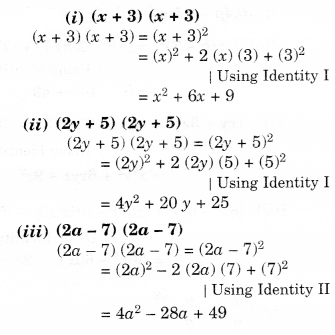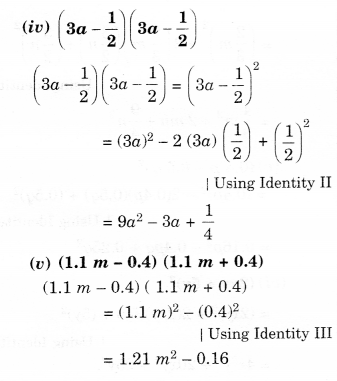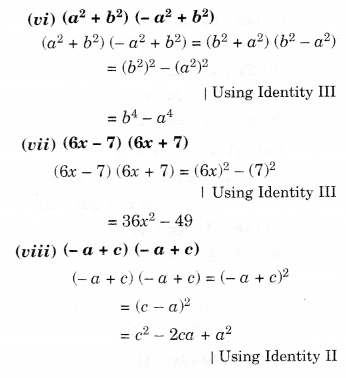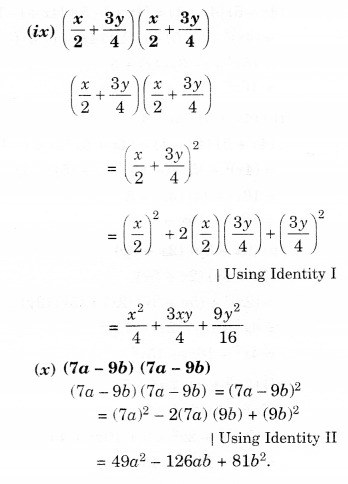Question 2.
Use the identity $$(x+a)(x+b)={ x }^{ 2 }+(a+b)x+ab$$ to find the following products:
(i) (x + 3) (x + 7)
(ii) (4x + 5) (4x + 1)
(iii) (4x – 5) (4x – 1)
(iv) (4x + 5) (4x – 1)
(v) (2x + 5y) (2x + 3y)
(vi) $$(2{ a }^{ 2 }+9)(2{ a }^{ 2 }+5)$$
(vii) (xyz – 4) (xyz – 2).
Solution.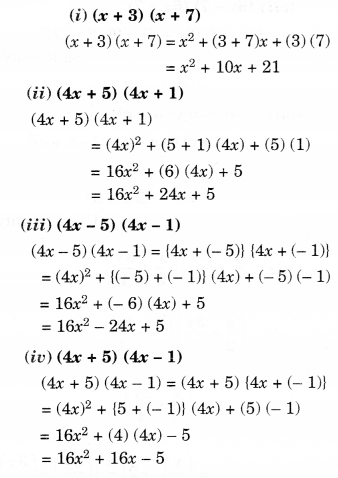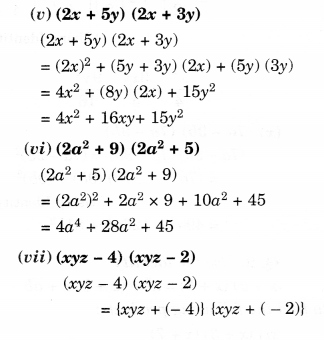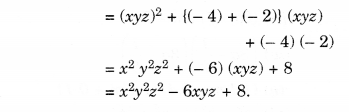Question 3.
Find the following squares by using the identities.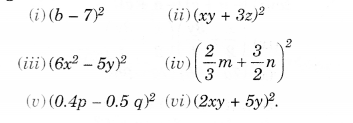Solution.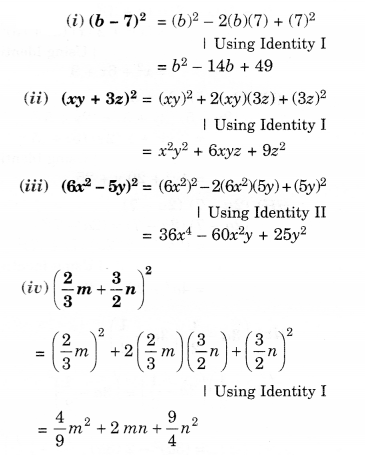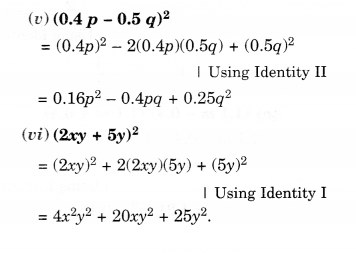Question 4.
Simplify: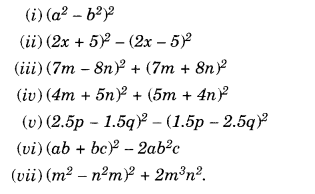Solution.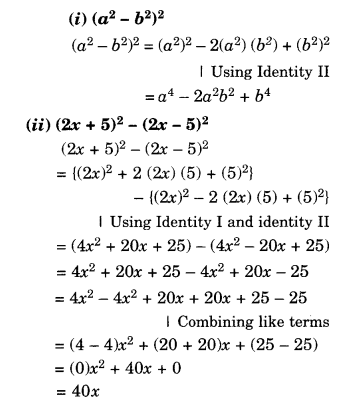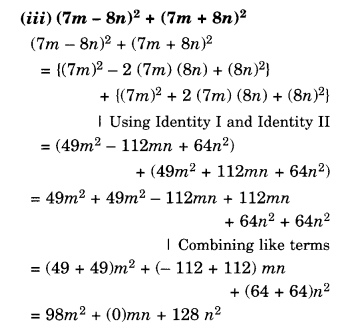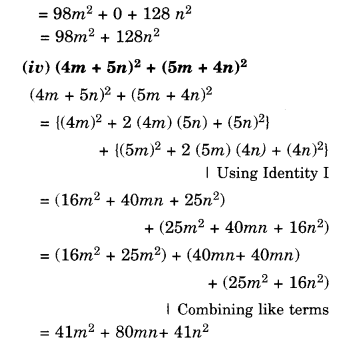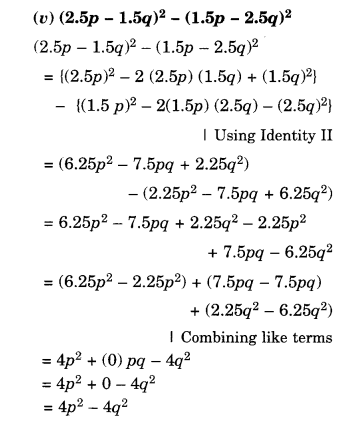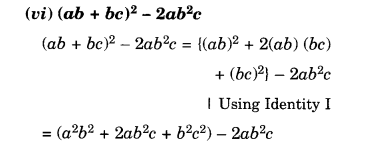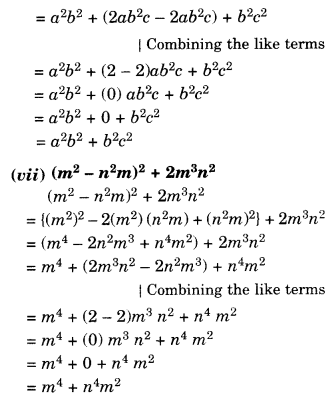Question 5.
Show that: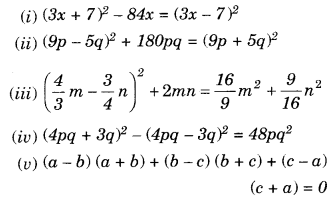Solution.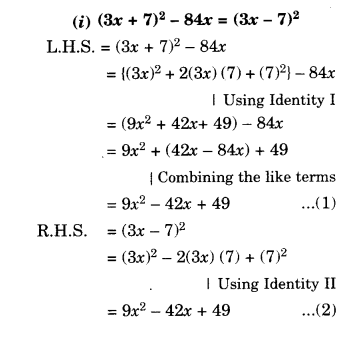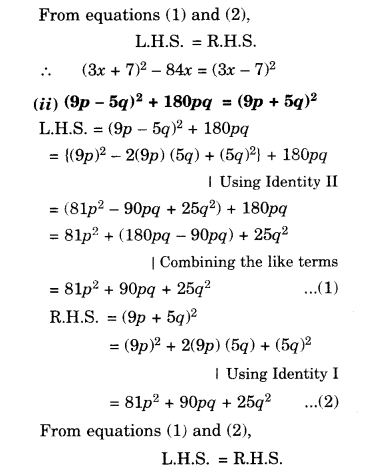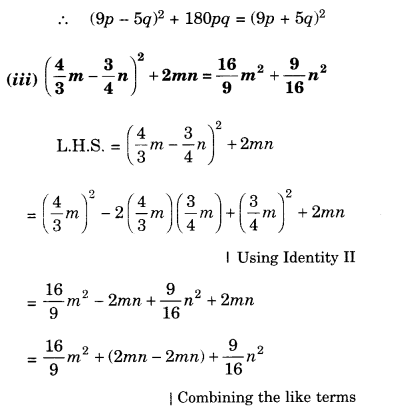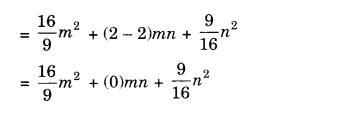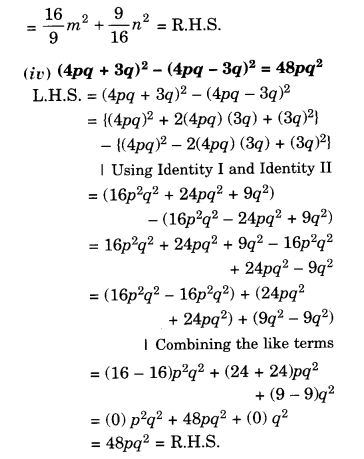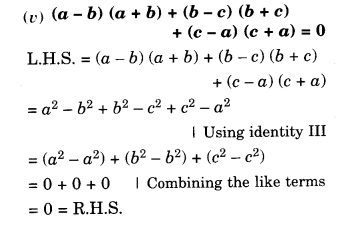Question 6.
Using identities, evaluate: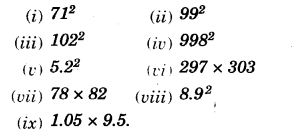Solution.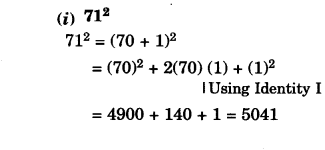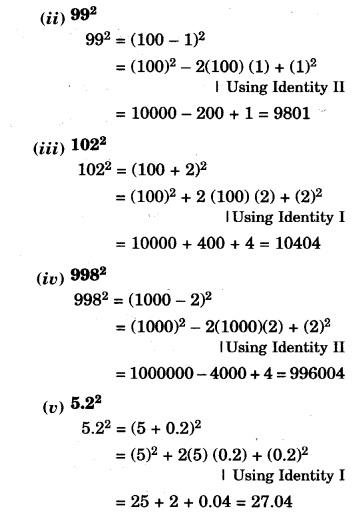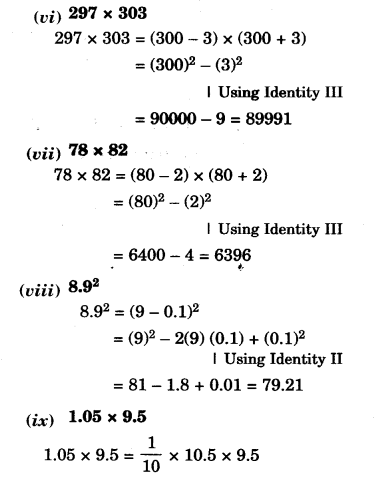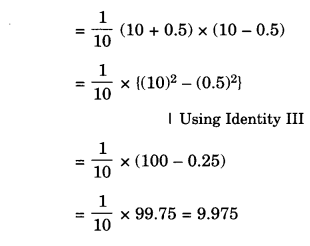Question 7.
Using $${ a }^{ 2 }-{ b }^{ 2 }=(a+b)(a-b)$$, find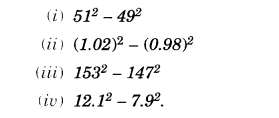Solution.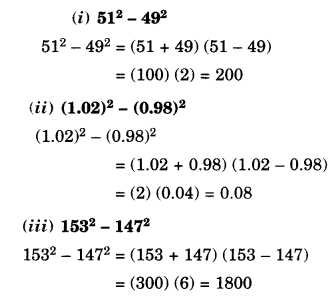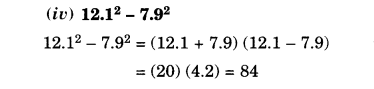Question 8.
Using $$(x+a)(x+b)={ x }^{ 2 }+(a+b)x+ab$$, find
(i) 103 x 104
(ii) 5.1 x 5.2
(iii) 103 x 98
(iv) 9.7 x 9.8
Solution.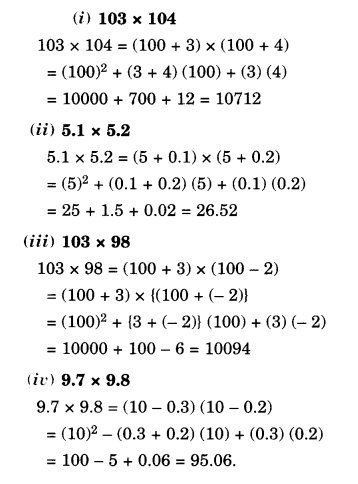We hope the NCERT Solutions for Class 8 Maths Chapter 9 Algebraic Expressions and Identities Ex 9.5 help you. If you have any query regarding NCERT Solutions for Class 8 Maths Chapter 9 Algebraic Expressions and Identities Ex 9.5, drop a comment below and we will get back to you at the earliest.